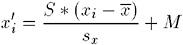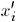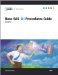# Statistical Computations: STANDARD Procedure

Standardizing values removes the location and scale attributes from a set of data. The formula to compute standardized values iswhereis a new standardized value S is the value of STD= M is the value of MEAN= x i is an observation s value x is a variable s mean s x is a variable s standard deviation.

PROC STANDARD calculates the mean ( x ) and standard deviation ( S x ) from the input data set. The resulting standardized variable has a mean of M and a standard deviation of S .

If the data are normally distributed, standardizing is also studentizing since the resulting data have a Student s t distribution.Base SAS 9.1 Procedures Guide, Volumes 1, 2, 3 and 4
ISBN: 1590472047
EAN: 2147483647
Year: 2004
Pages: 142